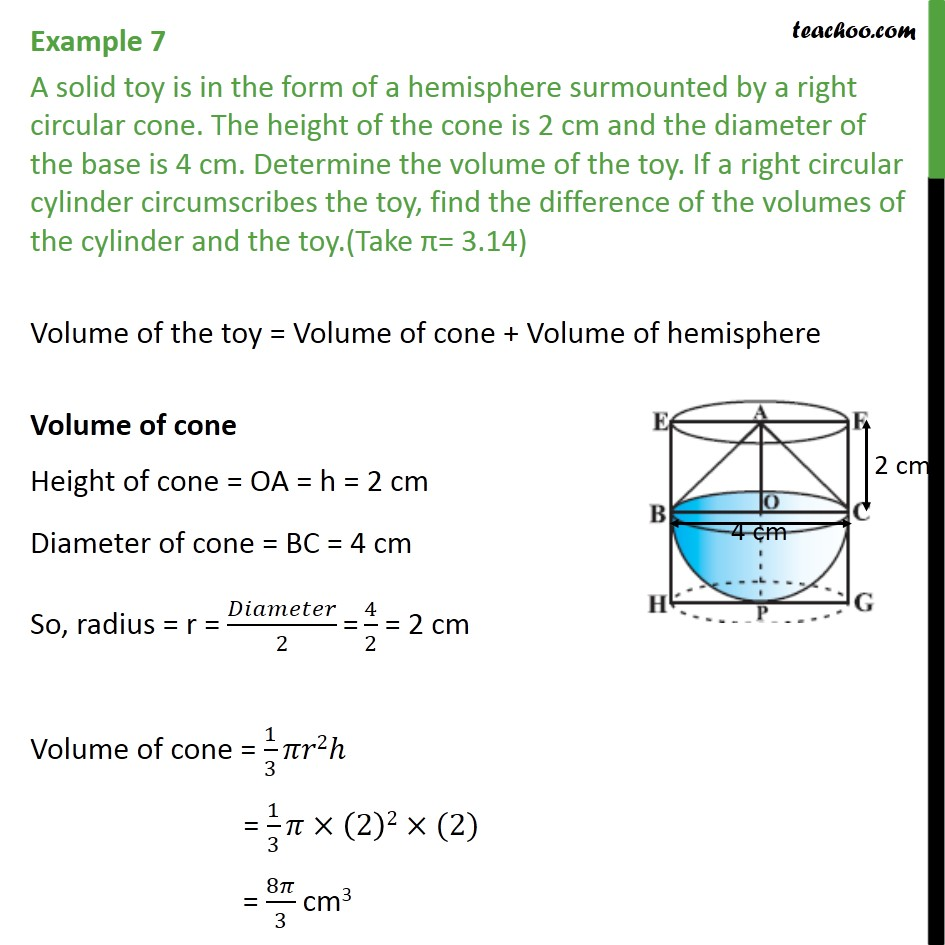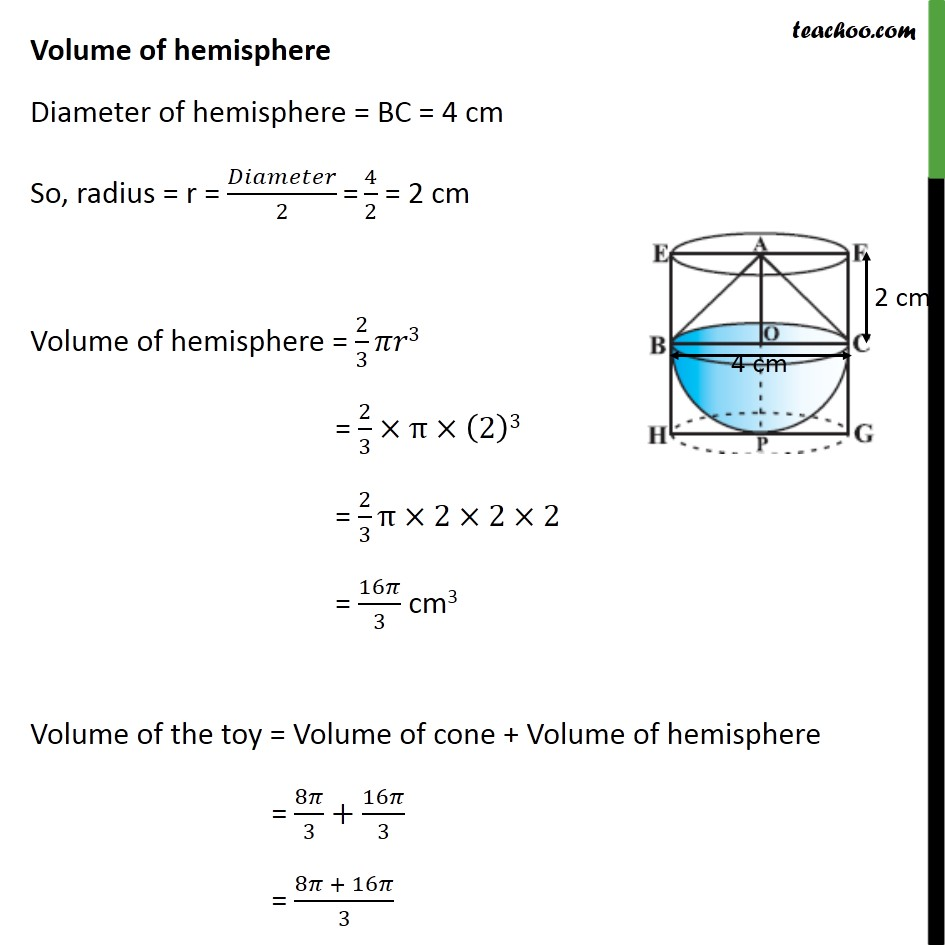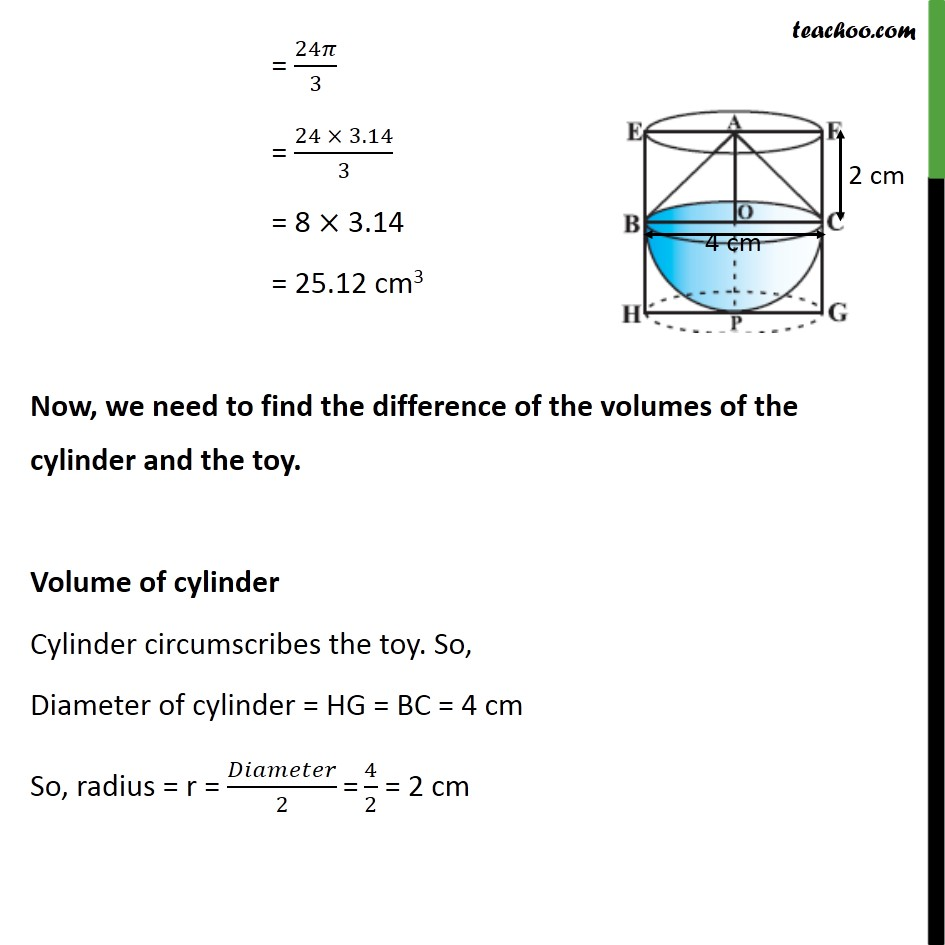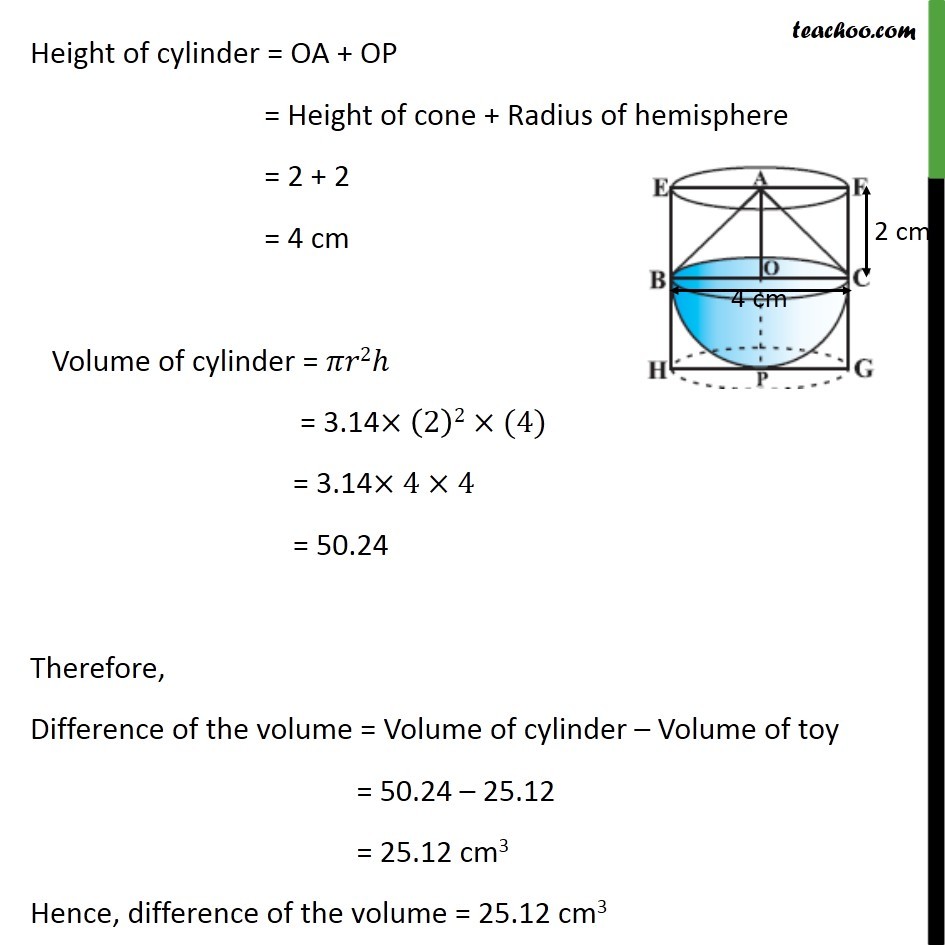Examples

Chapter 12 Class 10 Surface Areas and Volumes
Serial order wiseLearn in your speed, with individual attention - Teachoo Maths 1-on-1 Class

### Transcript

Example 7 A solid toy is in the form of a hemisphere surmounted by a right circular cone. The height of the cone is 2 cm and the diameter of the base is 4 cm. Determine the volume of the toy. If a right circular cylinder circumscribes the toy, find the difference of the volumes of the cylinder and the toy.(Take = 3.14) Volume of the toy = Volume of cone + Volume of hemisphere Volume of cone Height of cone = OA = h = 2 cm Diameter of cone = BC = 4 cm So, radius = r = /2 "=" 4/2 = 2 cm Volume of cone = 1/3 2 = 1/3 (2)2 (2) = 8 /3 cm3 Volume of hemisphere Diameter of hemisphere = BC = 4 cm So, radius = r = /2 "=" 4/2 = 2 cm Volume of hemisphere = 2/3 3 = 2/3 (2)3 = 2/3 2 2 2 = 16 /3 cm3 Volume of the toy = Volume of cone + Volume of hemisphere = 8 /3+16 /3 = (8 + 16 )/3 = 24 /3 = (24 3.14)/3 = 8 3.14 = 25.12 cm3 Now, we need to find the difference of the volumes of the cylinder and the toy. Volume of cylinder Cylinder circumscribes the toy. So, Diameter of cylinder = HG = BC = 4 cm So, radius = r = /2 "=" 4/2 = 2 cm Height of cylinder = OA + OP = Height of cone + Radius of hemisphere = 2 + 2 = 4 cm Volume of cylinder = 2 = 3.14 (2)2 (4) = 3.14 4 4 = 50.24 Therefore, Difference of the volume = Volume of cylinder Volume of toy = 50.24 25.12 = 25.12 cm3 Hence, difference of the volume = 25.12 cm3